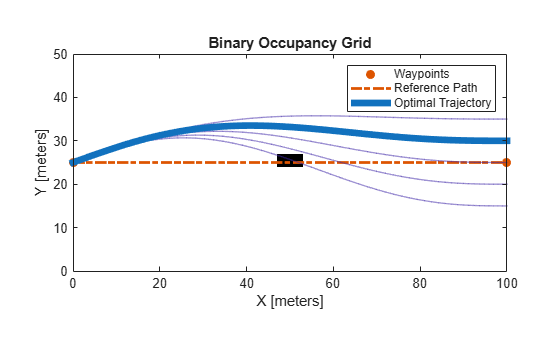# show

Visualize trajectories

## Syntax

``show(planner)``
``show(planner,Name,Value)``
``axHandle = show(planner)``

## Description

````show(planner)` visualizes the reference path and trajectory from the candidates generated by the `plan` function. The trajectory is shown as a line plot. The plot also includes datatip mode, which can be used to visualize the feasibility vector and index of the trajectory from the TrajectoryList property.```

example

````show(planner,Name,Value)` specifies additional options using one or more `Name,Value` pair arguments.```
````axHandle = show(planner)` returns the axes handle of the figure used to plot the trajectory.```

## Examples

collapse all

This example shows how to plan an optimal trajectory using a `trajectoryOptimalFrenet` object.

Create and Assign Map to State Validator

Create a state validator object for collision checking.

`stateValidator = validatorOccupancyMap;`

Create an obstacle grid map.

```grid = zeros(50,100); grid(24:26,48:53) = 1;```

Create a `binaryOccupancyMap` with the grid map.

`map = binaryOccupancyMap(grid);`

Assign the map and the state bounds to the state validator.

```stateValidator.Map = map; stateValidator.StateSpace.StateBounds(1:2,:) = [map.XWorldLimits; map.YWorldLimits];```

Plan and Visualize Trajectory

Create a reference path for the planner to follow.

`refPath = [0,25;100,25];`

Initialize the planner object with the reference path, and the state validator.

`planner = trajectoryOptimalFrenet(refPath,stateValidator);`

Assign longitudinal terminal state, lateral deviation, and maximum acceleration values.

```planner.TerminalStates.Longitudinal = 100; planner.TerminalStates.Lateral = -10:5:10; planner.FeasibilityParameters.MaxAcceleration = 10;```

Specify the deviation offset value close to the left lateral terminal state to prioritize left lane changes.

`planner.DeviationOffset = 5;`

Trajectory Planning

Initial cartesian state of vehicle.

`initCartState = [0 25 pi/9 0 0 0];`

Convert cartesian state of vehicle to Frenet state.

`initFrenetState = cart2frenet(planner,initCartState);`

Plan a trajectory from initial Frenet state.

`plan(planner,initFrenetState);`

Trajectory Visualization

Visualize the map and the trajectories.

```show(map) hold on show(planner,'Trajectory','all')```This example shows how to partition the longitudinal terminal states in optimal trajectory planning using a `trajectoryOptimalFrenet` object.

Create and Assign Map to State Validator

Create a state validator object for collision checking.

`stateValidator = validatorOccupancyMap; `

Create an obstacle grid map.

```grid = zeros(50,100); grid(25:27,28:33) = 1; grid(16:18,37:42) = 1; grid(29:31,72:77) = 1;```

Create a `binaryOccupancyMap` with the grid map.

`map = binaryOccupancyMap(grid);`

Assign the map and the state bounds to the state validator.

```stateValidator.Map = map; stateValidator.StateSpace.StateBounds(1:2,:) = [map.XWorldLimits; map.YWorldLimits];```

Plan and Visualize Trajectory

Create a reference path for the planner to follow.

`refPath = [0,25;30,30;75,20;100,25];`

Initialize the planner object with the reference path, and the state validator.

`planner = trajectoryOptimalFrenet(refPath,stateValidator);`

Assign longitudinal terminal state, lateral deviation, and maximum acceleration values.

```planner.TerminalStates.Longitudinal = 100; planner.TerminalStates.Lateral = -5:5:5; planner.FeasibilityParameters.MaxAcceleration = 10;```

Assign the number of partitions for the longitudinal terminal state.

`planner.NumSegments = 3;`

Trajectory Planning

Initial Frenet state of vehicle.

`initFrenetState = zeros(1,6);`

Plan a trajectory from initial Frenet state.

`plan(planner,initFrenetState);`

Trajectory Visualization

Visualize the map and the trajectories.

```show(map) hold on show(planner,'Trajectory','all') hold on```

Generate Lane Boundaries

Calculate end of reference path as Frenet state.

`refPathEnd = cart2frenet(planner,[planner.Waypoints(end,:) 0 0 0 0]);`

Calculate lane offsets on both sides of the lateral terminal states with half lane width value.

`laneOffsets = unique([planner.TerminalStates.Lateral+2.5 planner.TerminalStates.Lateral-2.5]);`

Calculate positions of lanes in Cartesian state.

```numLaneOffsets = numel(laneOffsets); xRefPathEnd = ceil(refPathEnd(1)); laneXY = zeros((numLaneOffsets*xRefPathEnd)+numLaneOffsets,2); xIndex = 0; for laneID = 1:numLaneOffsets for x = 1:xRefPathEnd laneCart = frenet2cart(planner,[x 0 0 laneOffsets(laneID) 0 0]); xIndex = xIndex + 1; laneXY(xIndex,:) = laneCart(1:2); end xIndex = xIndex + 1; laneXY(xIndex,:) = NaN(1,2); end```

Plot lane boundaries.

`plot(laneXY(:,1),laneXY(:,2),'LineWidth',0.5,'Color',[0.5 0.5 0.5],'DisplayName','Lane Boundaries','LineStyle','--')`## Input Arguments

collapse all

Optimal trajectory planner in Frenet space, specified as a `trajectoryOptimalFrenet` object.

### Name-Value Arguments

Specify optional comma-separated pairs of `Name,Value` arguments. `Name` is the argument name and `Value` is the corresponding value. `Name` must appear inside quotes. You can specify several name and value pair arguments in any order as `Name1,Value1,...,NameN,ValueN`.

Example: `'Trajectory','all'`

Axes to plot trajectory, specified as the comma-separated pair consisting of `'Parent'` and either an `Axes` or `UIAxes` object. See `axes` or `uiaxes`.

Trajectory display option, specified as the comma-separated pair consisting of `'Trajectory'` and either `'optimal'` or `'all'`.

Reference path display option, specified as the comma-separated pair consisting of `'ReferencePath'` and either `'on'` or `'off'`.

Trajectory color display option, specified as the comma-separated pair consisting of `'TrajectoryColor'` and one of the following:

• `'acceleration'`

• `'cost'`

• `'velocity'`

• `'none'`

Set this property to display the specified trajectory as a color-gradient along the specified path.

## Output Arguments

collapse all

Axes handle used to plot trajectory, returned as either an `axes`, or `uiaxes` object.